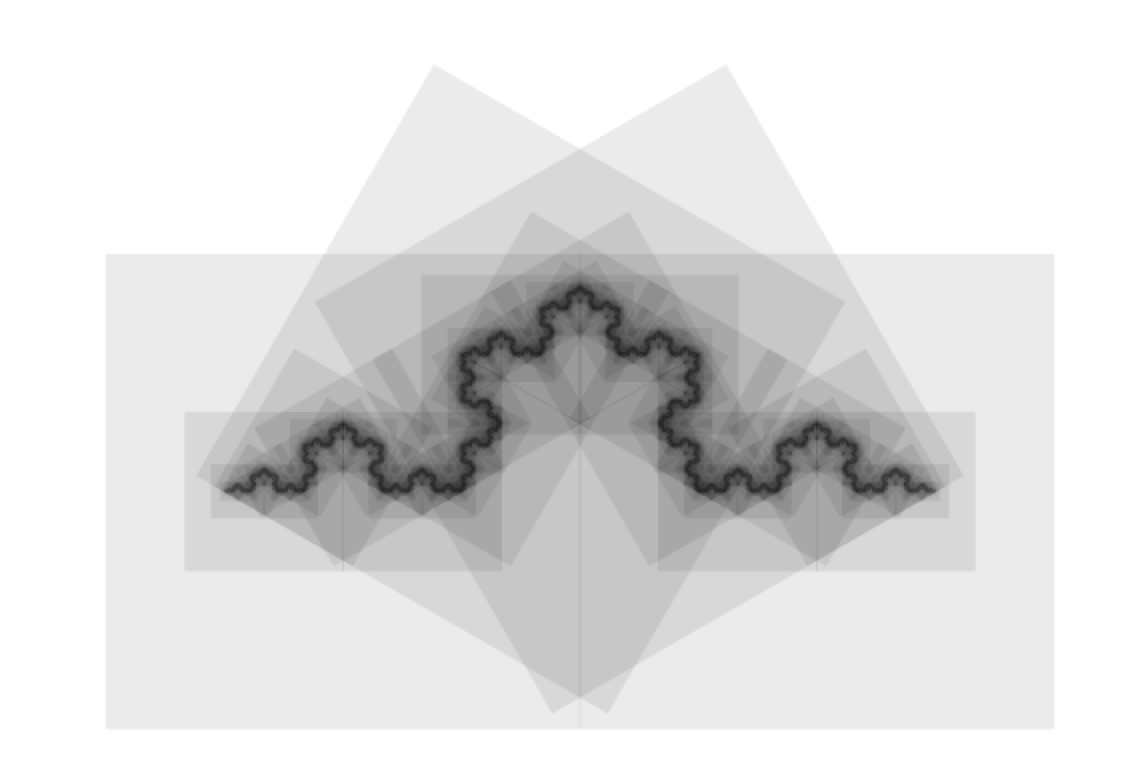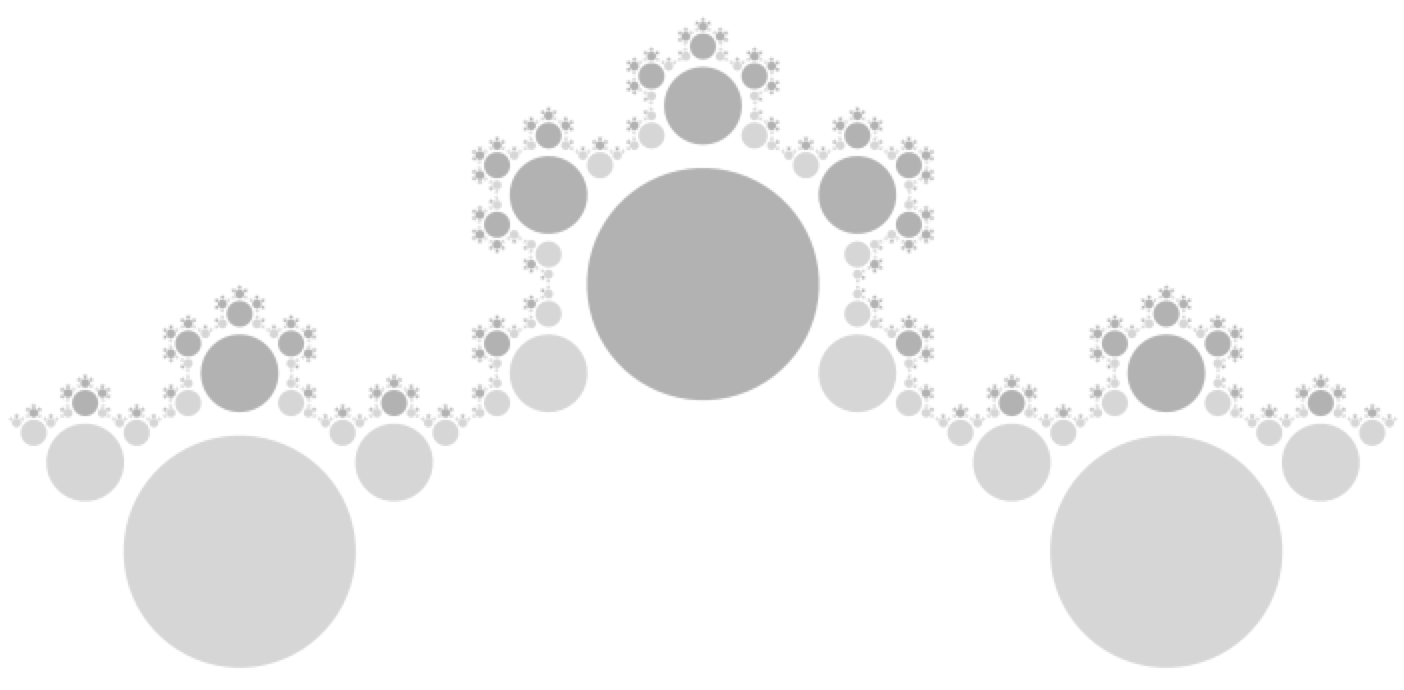## Koch Snowflakes and CFDG

CFDG ('Context-Free Design Grammer', but don't let that put you off) is a fun little program that allows you to build up complex pictures using elementary shapes, often repeated thousands or even millions of times. It makes it very easy to do recursive shapes, and also random-branching (for instance, you can specify that shape 'A' does one thing randomly  99 times out of 100 and something else the other time). It has an online gallery of user submitted pictures and the code used to generate them.

What I really like about CDFG is the organic quality some of the pictures have, and also some of the complexity that's possible with a very small amount of code. It's fun to look through the most popular images and try to guess how long the code is just by looking at the output.

### Koch Snowflake

Because CDFG is good at recursive code, it's easy to make certain kinds of fractals. For example, one side of a koch snowflake can be made from repetitions of a simple basic shape:To make this in CDFG, we could use the following code:

`startshape spikesqrt3over4 = sqrt(3)/4one_third = 1/3shape line{ SQUARE[s 1 0.01]}shape spike{line[x -1]line[r 60 x -0.25 y  sqrt3over4]line[r -60 x 0.25 y  sqrt3over4]line[x 1]}`

Here we define two shapes, 'line' which draws a line (we're actually using a squished square, scaled 1 in the x dimension and 0.01 in the y) and 'spike', which draws four lines (the x and y values specify relative locations and r is a rotation in degrees). To generate the fractal we just need to make one change to the line function to call spike again reduced in size by a factor of 3:

`startshape spikesqrt3over4 = sqrt(3)/4one_third = 1/3shape line{ SQUARE[s 1 0.01] spike[s one_third]}shape spike{line[x -1]line[r 60 x -0.25 y  sqrt3over4]line[r -60 x 0.25 y  sqrt3over4]line[x 1]}`

Which gives us the following:There's a small problem with this approach: when we scale the next generation of the shape, it scales in all dimensions, including the line width, making later generations harder to see and so we don't get a good view of what we really want to see, which is what the final generations tend to.

What's fun about the code above is that, since we're only interested in the final generations, and since the final generations are going to eventually be ~1pixel big, it really doesn't matter what shape we use in the line shape. It helps to make the shape transparent (we can use the 'a' (alpha) parameter for that) so that we don't block subsequent generations, but it doesn't have to be a line at all. For example, if we replace the SQUARE[s 1 0.01] with CIRCLE[a -0.9] we get the following:We can use all kinds of shapes in the line shape to produce different early generations, but all tending towards the same snowflake perimeter.
 SQUARE[s 2 a -0.9]TRIANGLE [s 1.5 alpha -0.9]CIRCLE [  s .5 alpha -0.8]CIRCLE [s .5 alpha -0.8 y -.288675]SQUARE[s 1 x 1 y -1 a -0.9]You can find my small selection of CFDG scripts here.